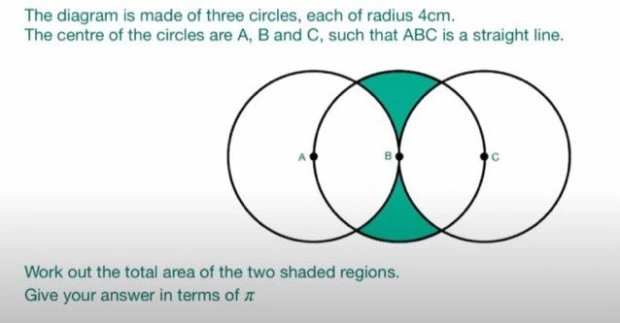# The Hardest GCSE Maths Question

22-05-2022

Bare in mind, this question was asked to 16 year olds in the UK during their final maths exam.

## Question## Solution

\begin{aligned} \text{Let N the intercection of circles A and B at the top} \\ AB = 4 \\ \text{Equilateral Triangle ABN - side length 4, internal angles all 60°}\\ \text{Area: } \frac{1}{2}(4)(4)\sin{60} = 4\sqrt{3}\\ \text{Notice that lines AN and AB form a segment of circle A: }\\ \frac{60}{360}(\pi 4^2) = \frac{8}{3}\pi\\ \text{Thus, we can get the area of the remainder of the circle-segment by finding the difference: } \\ \frac{8}{3}\pi-4\sqrt{3} \end{aligned}

OK I got bored here Lol but I think you see where I'm headed. Not too complex but tricky to see where to start May revisit!

I also (occasionally) write an email newsletter! You can subscribe here if you're interested: